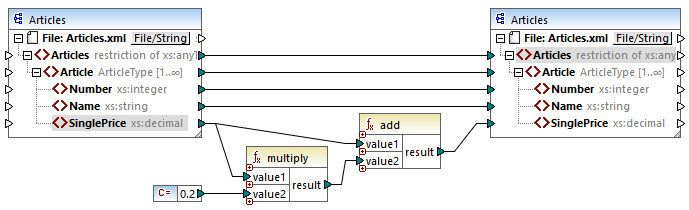# Altova MapForce 2022 Enterprise Edition

## core | math functions

Math functions are used to perform basic mathematical operations on data. Note that they cannot be used to perform computations on durations or datetime values.

Most math functions take two input parameters (value1, value2) that are operands of the mathematical operation. The input values are automatically converted to decimal type for further processing. The result of math functions is also of decimal type.The example shown above adds 20% sales tax to each of the articles mapped to the target component.